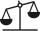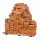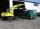# Brick weight

Half the weight of a brick plus 20 pounds is equal to 1/3 the weight of the brick plus 30 pounds. How much does the brick weigh?

Result

x =  60 lb

#### Solution:

x/2 + 20 = x/3 + 30

x = 60

x = 60

Calculated by our simple equation calculator.

Leave us a comment of example and its solution (i.e. if it is still somewhat unclear...):Be the first to comment!#### To solve this example are needed these knowledge from mathematics:

Need help calculate sum, simplify or multiply fractions? Try our fraction calculator. Do you have a linear equation or system of equations and looking for its solution? Or do you have quadratic equation?

## Next similar examples:

1. BrickIsosceles scale has on one side all brick and second weight 1 kg and 1/4 of brick. The balance is in equilibrium. What is the weight of a brick?
2. Two bricksOne brick weighs 1kg and half a brick, how many weigh two bricks?
3. Grandmother and grandfatherGrandmother baked cakes. Grandfather ate half, then quarter of the rest ate Peter and Paul ate half of rest. For parents left 6 cakes. How many cakes maked the grandmother?
4. Fraction and a decimalWrite as a fraction and a decimal. One and two plus three and five hundredths
5. FlourKim needs 3/4 cup of flour to make 12 cookies. How much flour would she need to make 60 cookies?
6. In fractionsAn ant climbs 2/5 of the pole on the first hour and climbs 1/4 of the pole on the next hour. What part of the pole does the ant climb in two hours?
7. Coal storageThe coal storage distribute received coal shipment within three days. The first day distribute third of the shipments, the second day of two-fifths of the rest and the third day 300 tons of coal. How many tons of coal distributed first and second day?
8. Simple equation 5Solve equation with fractions: X × 3/8 = 1/2
9. Fraction to decimalWrite the fraction 3/22 as a decimal.
10. Mixed2improperWrite the mixed number as an improper fraction. 166 2/3
11. ZdeněkZdeněk picked up 15 l of water from a 100-liter full-water barrel. Write a fraction of what part of Zdeněk's water he picked.
12. Equation 20In given equation: 8/9-4/5=2/9+x, find x
13. Lengths of the poolMiguel swam 6 lengths of the pool. Mat swam 3 times as far as Miguel. Lionel swam 1/3 as far as Miguel. How many lengths did mat swim?
14. Forest nurseryIn the forest nursery after winter, they found that 1/10 stems died out of them. For them, they land 193 new spruces. How many spruces are in the forest nursery?
15. TreesFrom the total number of trees in the orchard, there are two-fifths pearls and apples are three eighty. The rest of the trees are 9 ceremonial. How many trees are in the set?
16. Find unknownFind unknown numerator: 4/8 + _/8 = 1
17. Equation 15Solve equation with variables on both sides: